# Diagonal theorem

A generic theorem generalizing the classical "sliding hump" method given by H. Lebesgue and O. Toeplitz, see [a3], and very useful in the proof of generalized fundamental theorems of functional analysis and measure theory.

Let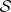be a commutative semi-group with neutral elementand with a triangular functional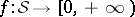, i.e.and. For each sequence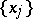inand each, one writes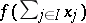for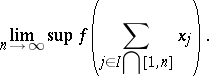The Mikusiński–Antosik–Pap diagonal theorem ([a1], [a4], [a5], [a6]) reads as follows. Letbe an infinite matrix (indexed by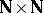) with entries in. Suppose that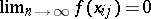,. Then there exist an infinite setand a set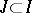such that

a)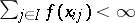,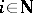; and

b)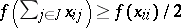,.

The following diagonal theorem is a consequence of the preceding one ([a1], [a6], [a8]): Letbe a commutative group with a quasi-norm, i.e.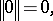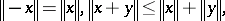and let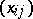be an infinite matrix insuch that for every increasing sequencein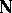there exists a subsequenceof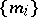such that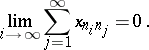Then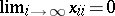.

Proofs involving diagonal theorems are characterized not only by simplicity but also by the possibility of further generalization. A great number of fundamental theorems in functional analysis and measure theory have been proven by means of diagonal theorems, such as (see, e.g., [a1], [a5], [a6], [a7], [a9]): the Nikodým convergence theorem; the Vitali–Hahn–Saks theorem; the Nikodým boundedness theorem; the uniform boundedness theorem (cf. Uniform boundedness); the Banach–Steinhaus theorem; the Bourbaki theorem on joint continuity; the Orlicz–Pettis theorem (cf. Vector measure); the kernel theorem for sequence spaces; the Bessaga–Pelczynski theorem; the Pap adjoint theorem; and the closed-graph theorem.

Rosenthal's lemma [a2] is closely related to diagonal theorems. Many related results can be found in [a1], [a2] [a5], [a6], [a9], where the method of diagonal theorems is used instead of the usually used Baire category theorem, which is equivalent with a weaker form of the axiom of choice.